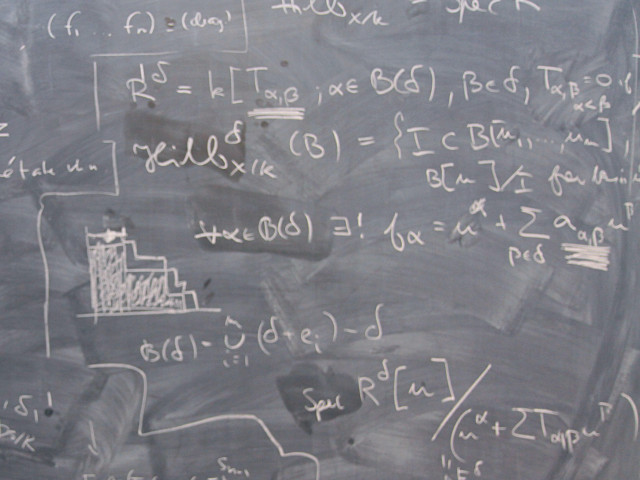SF2522 Computational Methods for Stochastic Differential Equations 7.5 credits

Beräkningsmetoder för stokastiska differentialekvationerAdvanced course on modeling, analysis and effecient computation of solutions of problems with stochastic phenomena in science and technology.

The course contains the basic mathematical theory of stochastic differential equations and optimal control as well as its application to real problems in financial mathematics, material science, fluid flow, broadcast networks, optimal design, optimal reconstruction and chemical reactions in cell biology.

Offering and execution

No offering selected

Select the semester and course offering above to get information from the correct course syllabus and course offering.

Content and learning outcomes

Course contents *

The course treats stochastic differential equations and their numerical solution, with applications in financial mathematics, turbulent diffusion, control theory and Monte Carlo methods. We discuss basic questions for solving stochastic differential equations, e.g. to determine the price of an option is it more efficient to solve the deterministic Black and Scholes partial differential equation or use a Monte Carlo method based on stochastics.

The course treats basic theory of stochastic differential equations including weak and strong approximation, efficient numerical methods and error estimates, the relation between stochastic differential equations and partial differential equations, stochastic partial differential equations, variance reduction.

Intended learning outcomes *

After completing this master level course the students will be able to model, analyze and efficiently compute solutions to problems including random phenomena in science and engineering. The student learns the basic mathematical theory for stochastic differential equations and optimal control and applies it to some real-world applications, including financial mathematics, material science, geophysical flow, radio networks, optimal design, optimal reconstruction, and chemical reactions in cell biology.

More precisely the goal of the course means that the student can:

• present some models in science and finance based on stochastic differential equations and evaluate methods to determine their solution,
• derive and use the correspondence between expected values of stochastic diffusion processes and solutions to certain deterministic partial differential equations,
• formulate, use and analyze the main numerical methods for stochastic differential equations, based on Monte Carlo stochastics and partial differential equations,
• present some stochastic and deterministic optimal control problems in science and finance using differential equations and Markov chains,
• formulate, use and analyze deterministic and stochastic optimal control problems using both differential equations constrained minimization and dynamic programming (leading to the Hamilton-Jacobi-Bellman nonlinear partial differential equation),
• derive the Black-Scholes equation for options in mathematical finance and analyze the alternatives to determine option prices numerically,
• determine and analyze reaction rate problems for stochastic differential equation with small noise using optimal control theory.

Course Disposition

No information inserted

Literature and preparations

Specific prerequisites *

The prerequisite for the course is linear algebra, calculus, differential equations, probability and numerics corresponding to the first three years at KTH.

Recommended prerequisites

No information inserted

Equipment

No information inserted

Literature

To be announced at least 4 weeks before course start at course home page

Examination and completion

A, B, C, D, E, FX, F

Examination *

• LABA - Laboratory, 3.5 credits, Grading scale: P, F
• TEN1 - Examination, 4.0 credits, Grading scale: A, B, C, D, E, FX, F

Based on recommendation from KTH’s coordinator for disabilities, the examiner will decide how to adapt an examination for students with documented disability.

The examiner may apply another examination format when re-examining individual students.

In this course all the regulations of the code of honor at the School of Computer science and Communication apply, see: http://www.kth.se/csc/student/hederskodex/1.17237?l=en_UK.

Other requirements for final grade *

The course includes lectures, collaborative homework problems and student presentations of their computations inspired by recent research papers.
The homework and projects give credit points for a final written exam.

Opportunity to complete the requirements via supplementary examination

No information inserted

Opportunity to raise an approved grade via renewed examination

No information inserted

Further information

Course web

Further information about the course can be found on the Course web at the link below. Information on the Course web will later be moved to this site.

Course web SF2522

SCI/Mathematics

Main field of study *

Mathematics, Technology

Education cycle *

Second cycle

No information inserted

Contact

Anders Szepessy (szepessy@kth.se)

Ethical approach *

• All members of a group are responsible for the group's work.
• In any assessment, every student shall honestly disclose any help received and sources used.
• In an oral assessment, every student shall be able to present and answer questions about the entire assignment and solution.

Supplementary information

The course is given every second year.